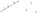Symmetry by plane

Determine the coordinates of a image of point A (3, -4, -6) at a symmetry that is determined by the plane x-y-4z-13 = 0

Result

x =  1
y =  -2
z =  2

Solution:Leave us a comment of this math problem and its solution (i.e. if it is still somewhat unclear...):Be the first to comment!To solve this verbal math problem are needed these knowledge from mathematics:

For Basic calculations in analytic geometry is helpful line slope calculator. From coordinates of two points in the plane it calculate slope, normal and parametric line equation(s), slope, directional angle, direction vector, the length of segment, intersections the coordinate axes etc.

Next similar math problems:

1. Vector - basic operationsThere are given points A [-9; -2] B [2; 16] C [16; -2] and D [12; 18] a. Determine the coordinates of the vectors u=AB v=CD s=DB b. Calculate the sum of the vectors u + v c. Calculate difference of vectors u-v d. Determine the coordinates of the vector w.
2. Third memberDetermine the third member of the AP if a4=93, d=7.5.
3. AS sequenceIn an arithmetic sequence is given the difference d = -3 and a71 = 455. a) Determine the value of a62 b) Determine the sum of 71 members.
4. Circle - AGFind the coordinates of circle and its diameter if its equation is: ?
5. Points collinearShow that the point A(-1,3), B(3,2), C(11,0) are col-linear.
6. LineLine p passing through A[-10, 6] and has direction vector v=(3, 2). Is point B[7, 30] on the line p?
7. LineIt is true that the lines that do not intersect are parallel?
8. Quadratic function 2Which of the points belong function f:y= 2x2- 3x + 1 : A(-2, 15) B (3,10) C (1,4)
9. Equation of circlefind an equation of the circle with indicated properties: a. center at (-3,5), diameter 20. b. center at origin and diameter 16.
10. Theorem proveWe want to prove the sentence: If the natural number n is divisible by six, then n is divisible by three. From what assumption we started?
11. Parametric equationFind the parametric equation of a line with y-intercept (0,-4) and a slope of -2.
12. Expressions 3If k(x+6)= 4x2 + 20, what is k(10)=?
13. GP membersThe geometric sequence has 10 members. The last two members are 2 and -1. Which member is -1/16?
14. AsymptoteWhat is the vertical asymptote of ?
15. TrucksThree lorries droved bricks. One drove n bricks at once, second m less bricks than the first and third 300 bricks more the first lorry. The first lorry went 4 times a day the largest went 3 times a day and the smallest 5 times a day. How many bricks br
16. ExpressionSolve for a specified variable: P=a+4b+3c, for a
17. Expression with powersIf x-1/x=5, find the value of x4+1/x4